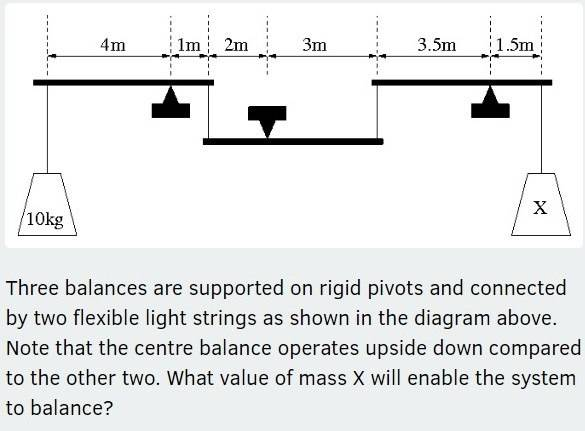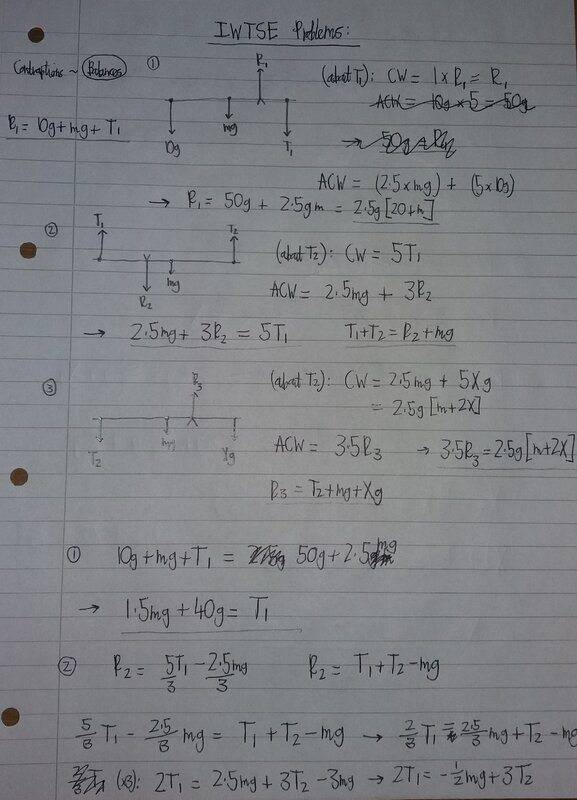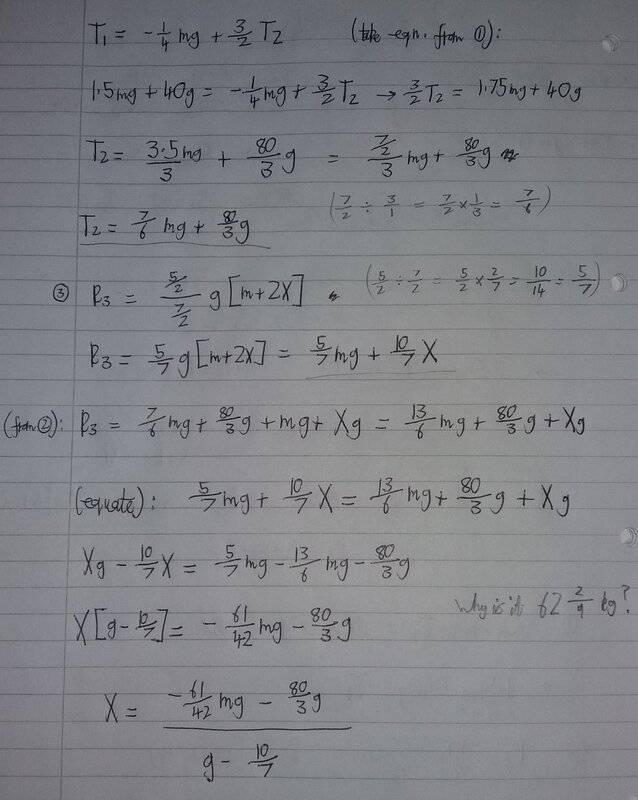# Moments Question (IWTSE.org) -- Three balances and two masses

TomK
Homework Statement:
Contraptions - Balances
Relevant Equations:
Moments EquationI think I have done this in an inefficient way. I assumed from the question that the three balances were of equal mass, though I'm not sure if I should have ignored their mass entirely. The correct answer is X = (62 + [2/9]) kg. Please help.

Last edited by a moderator:

Gold Member
Among the three balances, first think of the left one. How much weight is there at 1m arm for 10kg weight at 4m arm ? Then think of the right one how much weight is there at 3.5m arm for X kg weight at 3.5m arm ? With these two results think of the center balance.

Homework Helper
Gold Member
2022 Award
Homework Statement:: Contraptions - Balances
Relevant Equations:: Moments Equation
I think I have done this in an inefficient way. I assumed from the question that the three balances were of equal mass, though I'm not sure if I should have ignored their mass entirely. The correct answer is X = (62 + [2/9]) kg. Please help.
Yes, you should take the beams as zero mass, otherwise you do not have enough information.
At the end you have an expression X(g-10/7). Because you have not included units throughout, I am not sure what this means, but it is dimensionally inconsistent. g is an acceleration; the 10/7 may or may not be dimensionless, but it is certainly not an acceleration.

Having arrived at clear proof of an algebraic error, I would apply 'binary chop': check an equation somewhere in the middle for dimensional consistency; if that is wrong, check one quarter of the way through, else check at the three quarters mark; etc.

Homework Helper
Gold Member
For this specific problem, I would not bother with calculating the reaction forces at the three fulcrums.
Using mechanical advantage should be sufficient, if allowed.

•Keith_McClary
TomK
Yes, you should take the beams as zero mass, otherwise you do not have enough information.
At the end you have an expression X(g-10/7). Because you have not included units throughout, I am not sure what this means, but it is dimensionally inconsistent. g is an acceleration; the 10/7 may or may not be dimensionless, but it is certainly not an acceleration.

Having arrived at clear proof of an algebraic error, I would apply 'binary chop': check an equation somewhere in the middle for dimensional consistency; if that is wrong, check one quarter of the way through, else check at the three quarters mark; etc.

Thank you for your advice. I redid the question, assuming the beams have zero mass, and I got the right answer.

•Delta2